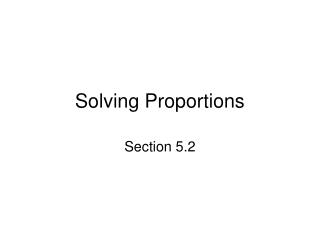DownloadDownload PresentationSolving Proportions

# Solving Proportions

Télécharger la présentation## Solving Proportions

- - - - - - - - - - - - - - - - - - - - - - - - - - - E N D - - - - - - - - - - - - - - - - - - - - - - - - - - -
##### Presentation Transcript

1. Solving Proportions Section 5.2

2. Objectives • Determine whether a proportion is true or false • Solve a proportion

3. Objective 1: Determine whether a proportion is true or false

4. Objective 1 • Proportions states two ratios are equal • Cross multiplication – checks whether proportion is true or false • Cross multiplication is shortcut for converting both fractions to have common denominator and checking that numerators match

5. Objective 1 • To check whether proportion is true or false • Check that ratios or rates have same units • Cross multiply • If cross products are equal, proportion is true

6. Objective 2:Solve a proportion

7. Objective 2 • If three numbers of proportion are given, you can find the fourth • To solve a proportion • Cross multiply • Do related division to find missing number